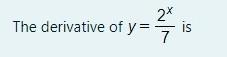### Create an Account

Home / Questions / 2x The derivative of y= y= is 7

# 2x The derivative of y= y= is 7

2x The derivative of y= y= is 7May 16 2021 View more View LessSubscribe To Get Solution